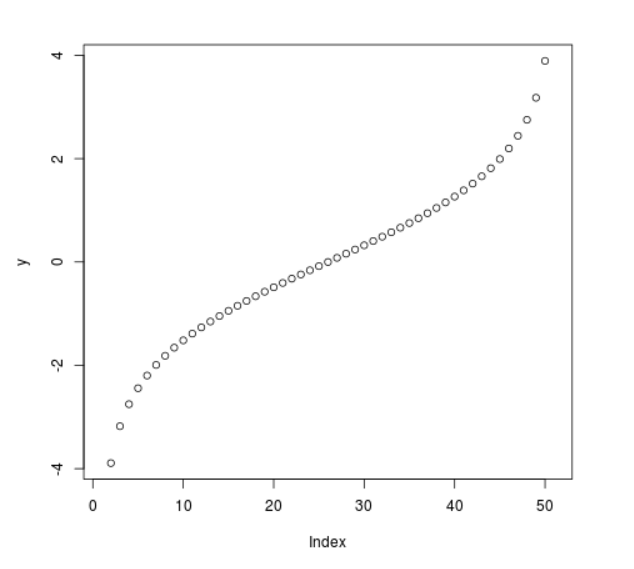# Compute value of Logistic Quantile Function in R Programming – qlogis() Function

• Last Updated : 25 Jun, 2020

`qlogis()` function in R Language is used to compute the value of logistic quantile function. It also creates a plot of the quantile function of logistic density distribution.

Syntax: qlogis(vec)

Parameters:
vec: x-values for normal density

Example 1:

 `# R program to compute value of``# logistic quantile function`` ` `# Creating x-values for density``x <``-` `seq(``0``, ``1``, by ``=` `0.2``)`` ` `# Calling qlogis() function``y <``-` `qlogis(x)``y`

Output:

```       -Inf -1.3862944 -0.4054651  0.4054651  1.3862944        Inf
```

Example 2:

 `# R program to compute value of``# logistic quantile function`` ` `# Creating x-values for density``x <``-` `seq(``0``, ``1``, by ``=` `0.02``)`` ` `# Calling qlogis() function``y <``-` `qlogis(x)`` ` `# Plot a graph``plot(y)`

Output:My Personal Notes arrow_drop_up